# Wave Graphs

Waves may be graphed as a function of time or distance. A single frequency wave will appear as a sine wave in either case. From the distance graph the wavelength may be determined. From the time graph, the period and frequency can be obtained. From both together, the wave speed can be determined.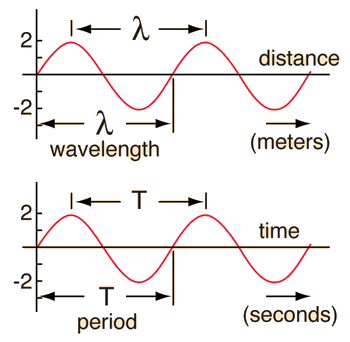Any of the wave parameters below can be changed. When you have finished entering data, click on the quantity you wish to calculate. The quantities will not be forced to be consistent until you click on the desired quantity.

### Wavelength = mFrequency = HzPeriod = s Amplitude = 2Wave velocity = m/s

 Traveling wave relationship Period and frequency
Index

Traveling wave concepts

 HyperPhysics***** Sound R Nave
Go Back

# Traveling Waves

Elasticity and a source of energy are the preconditions for periodic motion, and when the elastic object is an extended body, then the periodic motion takes the form of traveling waves.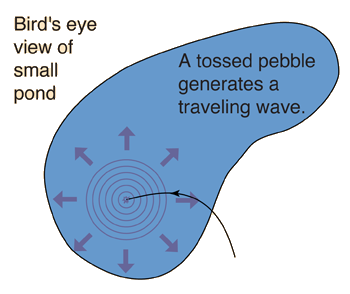A pond has an equilibrium level, and gravity serves as a restoring force. When work is done on the surface to disturb its level, a transverse wave is produced.A disturbance of the air pressure at a single point produces a spherical traveling pressure wave (sound). A sound wave in air is a longitudinal wave.

 Traveling wave relationship Wave plot example
Index

Traveling wave concepts

 HyperPhysics***** Sound R Nave
Go Back

# Waves on a Pond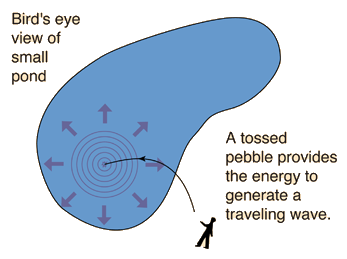### A pebble thrown into a pond will produce concentric circular ripples which move outward from the point of impact. If a fishing float is in the water, the float will bob up and down as the wave moves by. This is a characteristic of transverse waves. Such waves obey the wave relationship.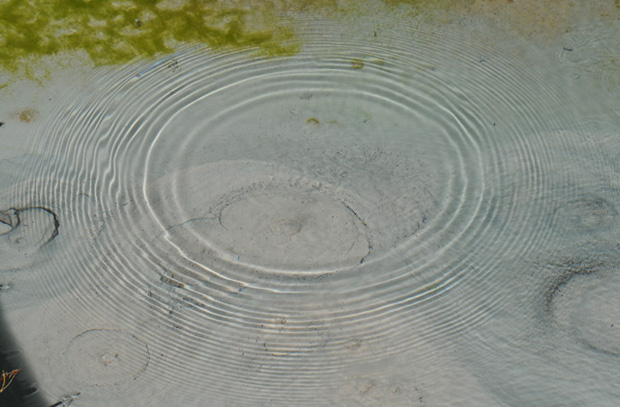This is an example of the transverse waves produced by bubbles rising from the bottom of this clear pool along the Icefields Parkway in Alberta, Canada. Although not exactly a circle, and not exactly a sine wave, these ripples show the tendency of surface disturbances to produce traveling waves in all directions from the source.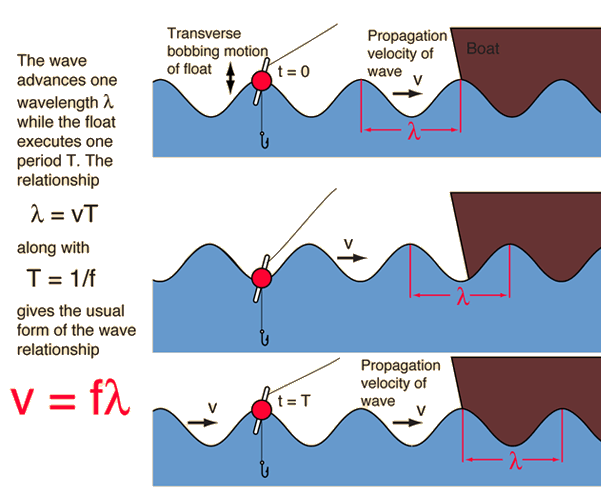The form of the dependence of the traveling wave parameters upon each other is a consequence of the basic motion equation and the definitions of the the terms which describe periodic motion. The description of water waves above is greatly oversimplified to demonstrate the nature of sinusoidal transverse waves. Waves on the ocean are much more complex, involving a circulation of the water as the wave passes so that the actual water motion is not just simple harmonic motion.

 Traveling wave relationship Wave plot example Simple harmonic motion
Index

Traveling wave concepts

 HyperPhysics***** Sound R Nave
Go Back